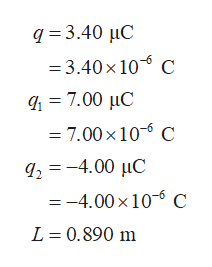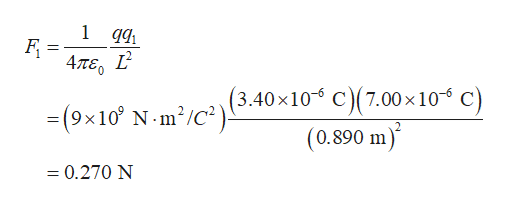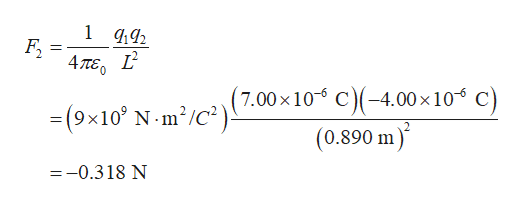Question
578 views

Three charged particles are located at the corners of an equilateral triangle as shown in the figure below (let q = 3.40 µC, and L = 0.890 m). Calculate the total electric force on the 7.00-µC charge.

Magnitude in Newtons?

Direction counterclockwise from the x-axis?

check_circle

Step 1

Consider the charges on the corners of the equilateral triangle as q, q1, and q2, the distance between the two charged particles as L, the electric force between the particles of charges q and q1 as F1 the electric force between the particles of charges q1 and q2 as F2.

The given values for the equilateral triangle are,help_outlineImage Transcriptioncloseq 3.40 uC = 3.40 x 10 C 7.00 C = 7.00 x 106 C q24.00 uC =-4.00 x 10 C L 0.890 m fullscreen
Step 2

Apply Coulomb’s law to calculate the electric force between the particles of charges q and q1.help_outlineImage Transcriptionclose1 qq 4ле, L (9x10 N m'/C3.40x10 C)(7.00 x 10 C (0.890 m) (3.40x106 C)(7.00 x 10 C) =0.270 N fullscreen
Step 3

Apply Coulomb’s law to calculate the electric force between the part...help_outlineImage Transcriptionclose1 2 = 4πε, 1 0 7.00 x 10 N m2/C2) C4.00 x 10 C) =(9x10 (0.890 m) --0.318 N fullscreen

### Want to see the full answer?

See Solution

#### Want to see this answer and more?

Solutions are written by subject experts who are available 24/7. Questions are typically answered within 1 hour.*

See Solution
*Response times may vary by subject and question.
Tagged in

### Physics